Programming a simple neural network

Though neural networks were considered to be of little use for a long time, the recent development of computing power and database size has proven otherwise. Since the revolution of machine learning in the last few years has been primarily driven by them, let’s dive right into the actual coding of neural nets.

Before coding, it can be useful to review the principles of neural nets to make sure we understand what we will be doing here. Thankfully, the work of coding has already been done by Milo Spencer-Harper, based upon the previous works of Andrew Trask. He guides us step by step into building a single-layer neural net and multiple-layer neural net, with crystal-clear coding and without using any machine learning library.

Since a single-layer neural net is of little use and the problem it solves can better be achieved through other methods, it is included here as a didactic step to better understand and learn to code multiple-layer neural nets.

In these two example, the complexity of the problem, and therefore the number of layers needed to solved it, consists in the number of columns of data to be taken into account. The single layer neural network solves the problem when one column of data is critical, the multiple-layers neural net (2 layers) when two columns are critical.

Single-layer neural net

In the first post, the building of a simple neural network is detailed through the following key steps synthesized here. The data set is a 3 columns matrix where only one column affects the results.  The single layer neural net is used to understand the direct influence this single column of data over the result.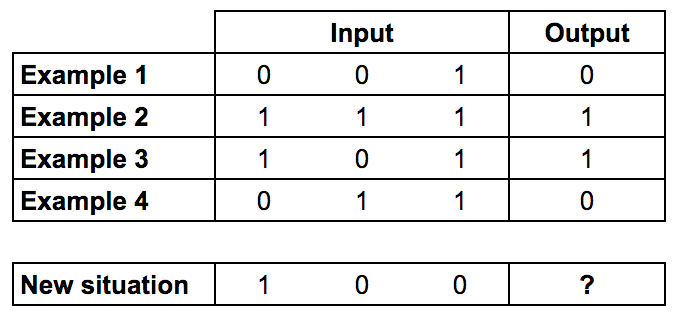Training data: Only the first column of input impacts the output

Since the code has been written in a previous version of Python, here are also included fully functional updated version for Python 3.6. Here is the light 9-line initial code for the single layer neural network.

Training Process

1. Take inputs, adjust with weights (positive or negative numbers), norm them through a sigmoid function
2. Calculate the error between the neuron’s output and the actual training data set
3. Adjust weights according to the error
4. Repeat 10,000 times

Calculate the neuron’s output

Sum the weighted input data into a sigmoid function to obtain normed results in the interval ]0;1[The final formula for the output of the neuron is: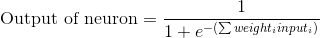Use the error weighted derivative = gradient descent

1. The neuron output from the sigmoid function indicates that if the output is close to 0 or 1, the data was close to the expected result
2. Close to 0 or 1, the derivative of the sigmoid function is almost flat
3. The adjustment to the weights based upon the derivative from the sigmoid function will therefore be very small when the neuron’s output is close to expected results or large when results differ from expectations

The sigmoid derivative equation is: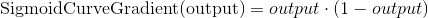So the final equation to adjust weights is: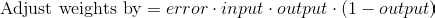Here is a fully functional version of the final code for the single-layer neural network with all details and comments, updated for Python 3.6.

Multiple-layer neural net

In the second post, the building of a multiple neural network is detailed through the following key steps reproduced. Bear in mind that such a neural network may be to complicated to solve simple problems and that it is best to understand nonlinear patterns, where the second layer of neurons can take combination of data inputs into account.

Here this two-layer neural net is used to understand how two columns in the data influence the results.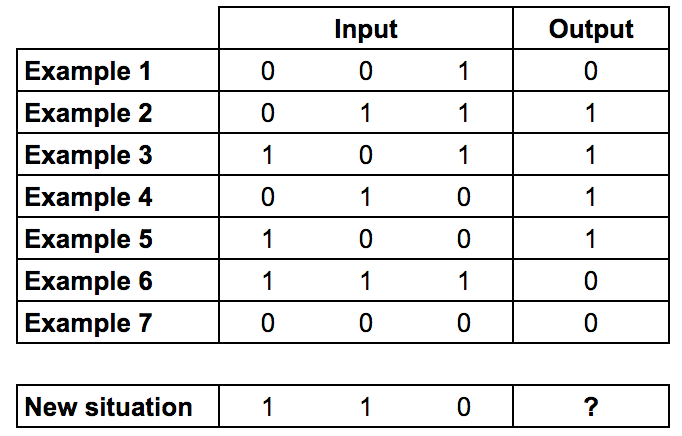Training data: The two first columns of input impact the output (XOR gate)

As Milo Spencer-Harper reminds us in his article, multiple layers are the source of the revolution in machine learning and artificial intelligence:

The process of adding more layers to a neural network, so it can think about combinations, is called deep learning.

The main difference in the code from the single-layer neural net is that the two layers influence the calculations for the error, and therefore the adjustment of weights. The errors from the second layer of neurons need to be propagated backwards to the first layer, this is called backpropagation.

Here is a fully functional version of the code for a two-layer neural network with all details and comments, updated for Python 3.6.

To sum up, in the following video, Siraj Raval goes over the detailed programming of a similar neural net (the original from Andrew Trask) in 4 minutes.

Attention: he uses an older version of Python, he also builds a 3-layer neural net, but the first layer is actually the input data without computation.

Image recognition by a deep neural net

Convolution: a neuron looks for patterns in a small portion (10×10 px) of an image (256×256 px), the process is repeated by moving this small area little by litte.

Pooling: The result of the convolution is computed as a point for each portion analyzed. By a similar step by step process, a small set of points are computed into values by choosing the maximum value (“max pooling”).

By reproducing the pooling process multiple times (100x), and feeding it to a neural net, it will compute how likely the initial image is recognized as a known category.

Autocoding

A small number of neurons (~2), the “hidden layer“, a bottleneck of neurons between two columns of multiple neurons (~10) is used to obtain output values z[n] that are the same as input values x[n].

Such results implies that a form a generalization is accomplished by the hidden layer, or rather, a form of encoded generalization, as the actual parameters of the bottleneck of neurons seems not so obvious to understand.

Final layer of neurons

As the neural net is trained with parameters and thresholds, the shape and corresponding equation of the sigmoid function is adapted to properly sort positive and negative results, by maximizing the probability of sorting examples properly.

Softmax

Instead of sorting by the maximum value and the corresponding category, the final output is an array of the most probable categories (~5 categories).

Dropout

The problems of neural nets is that they can get blocked in local maximum areas. To prevent this, at each computation, one neuron is deactivated to check if its behavior is skewing the neural net. At each new computation another is shut down, or dropped out, to check all neurons.

Thanks to wider neural networks, neural nets can avoid being jammed into local maximum as they can analyze local maximum through more parameters.

Boltzmann machine

Modeling biological neurons

Neural nets are modeled upon real biological neurons, which have the following characteristics:

1. All or none: the input from each entry in the neural net is either O or 1, the output is also 0 or 1
2. Cumulative influence: the influence of various input neurons accumulates to produce the final output result, if a certain threshold is reached
3. Synaptic weight: each input neuron is given a weight depending on its importance

Characteristics that are not modeled

Several characteristics of biological neurons are not modeled in neural nets, as their purpose is not clearly understood in brain information processing.

• Real neurons also have a refractory period when they do not respond to stimuli after firing.
• In a real neuron, the resulting output can go to either one (of a few) axonal bifurcations.
• The timing of the various inputs in the dendritic tree is not well understood in the resulting pulse in the axon.

Neural net principles

In a neural net, the resulting vector z, is a function of the different inputs x[n], the weights w[n] and the thresholds t[n]. A neural net is therefore a function approximator.

By comparing a known desired results vector (such as the content of a picture) with the actual output vector, a performance function can be determined to know how well the neural net is performing.

To simplify the performance function, thresholds are eliminated by adding an extra weight w that nullify the threshold, and the step function resulting in {0, 1} values is smoothed to a sigmoid function resulting in the [0, 1] interval.Backpropagation

Backpropagation is the name of the algorithm generally used to train a neural net.

Varying the weights little by little and with a certain randomization allows the performance function to measure if progress is being made or not, and to improve the weighing accordingly to progress towards an optimal performance.

The amount of computation of the performance function is linearly increased by the depth and squared by the width of the net.